Mrpt  1.1.1
Mrpt Class Reference

## Public Member Functions

Constructors

The constructor does not actually build the index. The building is done by the function grow() which has to be called before queries can be made. There are two different versions of the constructor which differ only by the type of the input data. The first version takes the data set as Ref to MatrixXf, which means that the argument can be either MatrixXf or Map<MatrixXf> (also certain blocks of MatrixXf may be accepted, see Eigen::Ref for more information). The second version takes a float pointer to an array containing the data set, and the dimension and the sample size of the data. There are also corresponding versions of all the member functions which take input data. In all cases the data is assumed to be stored in column-major order such that each data point is stored contiguously in memory. In all cases no copies are made of the original data matrix.

Mrpt (const Eigen::Ref< const Eigen::MatrixXf > &X_)

Mrpt (const float *X_, int dim_, int n_samples_)

Normal index building.

Build a normal (not autotuned) index.

void grow (int n_trees_, int depth_, float density_=-1.0, int seed=0)

Autotuned index building

Builds an index by autotuning such that the parameters giving the fastest query time at the target recall level are found. If the target recall level is not reached at all, then an index giving the highest recall level is built. The parameters() function can be used to retrieve these optimal parameter values and the estimated query time and the estimated recall. There is a version which uses a separate set of test queries (grow), and a version which samples a test set from the data set (grow_autotune).

void grow (double target_recall, const Eigen::Ref< const Eigen::MatrixXf > &Q, int k_, int trees_max=-1, int depth_max=-1, int depth_min_=-1, int votes_max_=-1, float density=-1.0, int seed=0)

void grow (double target_recall, const float *Q, int n_test, int k_, int trees_max=-1, int depth_max=-1, int depth_min_=-1, int votes_max_=-1, float density=-1.0, int seed=0, const std::vector< int > &indices_test={})

void grow_autotune (double target_recall, int k_, int trees_max=-1, int depth_max=-1, int depth_min_=-1, int votes_max_=-1, float density_=-1.0, int seed=0, int n_test=100)

Mrpt_Parameters parameters () const

bool is_autotuned () const

Autotuned index building without preset recall level

Build an autotuned index. This version does not require prespecifying a target recall level, but an index generated by this function can be used to subset different indices with different recall levels. This is done by subset(). The function optimal_parameters() can be used to retrieve a pareto frontier of optimal parameters. There is a version which uses a separate set of test queries (grow), and a version which samples a test set from the data set (grow_autotune).

void grow (const float *data, int n_test, int k_, int trees_max=-1, int depth_max=-1, int depth_min_=-1, int votes_max_=-1, float density_=-1.0, int seed=0, const std::vector< int > &indices_test={})

void grow (const Eigen::Ref< const Eigen::MatrixXf > &Q, int k_, int trees_max=-1, int depth_max=-1, int depth_min_=-1, int votes_max_=-1, float density_=-1.0, int seed=0)

void grow_autotune (int k_, int trees_max=-1, int depth_max=-1, int depth_min_=-1, int votes_max_=-1, float density_=-1.0, int seed=0, int n_test=100)

Mrpt subset (double target_recall) const

Mrptsubset_pointer (double target_recall) const

std::vector< Mrpt_Parametersoptimal_parameters () const

Approximate k-nn search

A query using a non-autotuned index. Finds k approximate nearest neighbors from a data set X for a query point q. Because the index is not autotuned, k and vote threshold are set manually. The indices of k nearest neighbors are written to a buffer out, which has to be preallocated to have at least length k. Optionally also Euclidean distances to these k nearest points are written to a buffer out_distances. If there are less than k points in the candidate set, -1 is written to the remaining locations of the output buffers.

void query (const float *data, int k, int vote_threshold, int *out, float *out_distances=nullptr, int *out_n_elected=nullptr) const

void query (const Eigen::Ref< const Eigen::VectorXf > &q, int k, int vote_threshold, int *out, float *out_distances=nullptr, int *out_n_elected=nullptr) const

Approximate k-nn search using autotuned index

Approximate k-nn search using an autotuned index. Finds k approximate nearest neighbors from a data set X for a query point q. Because the index is autotuned, no parameters other than a query point and an output are required: k is preset, and the optimal vote count is used automatically. The indices of k nearest neighbors are written to a buffer out, which has to be preallocated to have at least length k. Optionally also the Euclidean distances to these k nearest points are written to a buffer out_distances. If there are less than k points in the candidate set, -1 is written to the remaining locations of the output buffers.

void query (const float *q, int *out, float *out_distances=nullptr, int *out_n_elected=nullptr) const

void query (const Eigen::Ref< const Eigen::VectorXf > &q, int *out, float *out_distances=nullptr, int *out_n_elected=nullptr) const

Utility functions

Saving and loading an index and checking if it is already constructed. Saving and loading work for both autotuned and non-autotuned indices, and load() retrieves also the optimal parameters found by autotuning. The same data set used to build a saved index has to be used to construct the index into which it is loaded.

bool save (const char *path) const

bool load (const char *path)

bool empty () const

## Friends

Friend declarations for test fixtures. Tests are located at https://github.com/vioshyvo/RP-test.

class MrptTest

class UtilityTest

## Exact k-nn search

Functions for fast exact k-nn search: find k nearest neighbors for a query point q from a data set X_. The indices of k nearest neighbors are written to a buffer out, which has to be preallocated to have at least length k. Optionally also the Euclidean distances to these k nearest points are written to a buffer out_distances. There are both static and member versions.

void exact_knn (const float *q, int k, int *out, float *out_distances=nullptr) const

void exact_knn (const Eigen::Ref< const Eigen::VectorXf > &q, int k, int *out, float *out_distances=nullptr) const

static void exact_knn (const float *q_data, const float *X_data, int dim, int n_samples, int k, int *out, float *out_distances=nullptr)

static void exact_knn (const Eigen::Ref< const Eigen::VectorXf > &q, const Eigen::Ref< const Eigen::MatrixXf > &X, int k, int *out, float *out_distances=nullptr)

## ◆ Mrpt() [1/2]

 Mrpt::Mrpt ( const Eigen::Ref< const Eigen::MatrixXf > & X_ )
inline
Parameters
 X_ Eigen ref to the data set, stored as one data point per column

## ◆ Mrpt() [2/2]

 Mrpt::Mrpt ( const float * X_, int dim_, int n_samples_ )
inline
Parameters
 X_ a float array containing the data set with each data point stored contiguously in memory dim_ dimension of the data n_samples_ number of data points

## ◆ empty()

 bool Mrpt::empty ( ) const
inline

Is the index is already constructed or not?

Returns
- is the index empty?

## ◆ exact_knn() [1/4]

 static void Mrpt::exact_knn ( const float * q_data, const float * X_data, int dim, int n_samples, int k, int * out, float * out_distances = nullptr )
inlinestatic
Parameters
 q_data pointer to an array containing the query point X_data pointer to an array containing the data set dim dimension of data n_samples number of points in a data set k number of neighbors searched for out output buffer (size = k) for the indices of k nearest neighbors out_distances optional output buffer (size = k) for the distances to k nearest neighbors

## ◆ exact_knn() [2/4]

 static void Mrpt::exact_knn ( const Eigen::Ref< const Eigen::VectorXf > & q, const Eigen::Ref< const Eigen::MatrixXf > & X, int k, int * out, float * out_distances = nullptr )
inlinestatic
Parameters
 q Eigen ref to a query point X Eigen ref to a data set k number of neighbors searched for out output buffer (size = k) for the indices of k nearest neighbors out_distances optional output buffer (size = k) for the distances to k nearest neighbors

## ◆ exact_knn() [3/4]

 void Mrpt::exact_knn ( const float * q, int k, int * out, float * out_distances = nullptr ) const
inline
Parameters
 q pointer to an array containing the query point k number of neighbors searched for out output buffer (size = k) for the indices of k nearest neighbors out_distances optional output buffer (size = k) for the distances to k nearest neighbors

## ◆ exact_knn() [4/4]

 void Mrpt::exact_knn ( const Eigen::Ref< const Eigen::VectorXf > & q, int k, int * out, float * out_distances = nullptr ) const
inline
Parameters
 q pointer to an array containing the query point k number of points searched for out output buffer (size = k) for the indices of k nearest neighbors out_distances optional output buffer (size = k) for the distances to k nearest neighbors

## ◆ grow() [1/5]

 void Mrpt::grow ( int n_trees_, int depth_, float density_ = -1.0, int seed = 0 )
inline

Build a normal index.

Parameters
 n_trees_ number of trees to be grown depth_ depth of the trees; in the set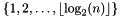, where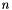is the number of data points density_ expected proportion of non-zero components in the random vectors; on the interval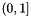; default value sets density to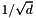, where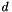is the dimension of the data seed seed given to a rng when generating random vectors; a default value 0 initializes the rng randomly with std::random_device

## ◆ grow() [2/5]

 void Mrpt::grow ( double target_recall, const Eigen::Ref< const Eigen::MatrixXf > & Q, int k_, int trees_max = -1, int depth_max = -1, int depth_min_ = -1, int votes_max_ = -1, float density = -1.0, int seed = 0 )
inline

Build an autotuned index.

Parameters
 target_recall target recall level; on the range [0,1] Q Eigen ref to the the test queries (col = data point, row = dimension). k_ number of nearest neighbors searched for trees_max number of trees grown; default value -1 sets this to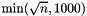, whereis the number of data points. depth_max maximum depth of trees considered when searching for optimal parameters; in the set, whereis the number of data points; default value -1 sets this to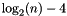, whereis the number of data points depth_min_ minimum depth of trees considered when searching for optimal parameters; in the set; a default value -1 sets this to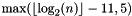votes_max_ maximum number of votes considered when searching for optimal parameters; a default value -1 sets this to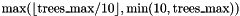density expected proportion of non-zero components in the random vectors; default value -1.0 sets this to, whereis the dimension of data seed seed given to a rng when generating random vectors; a default value 0 initializes the rng randomly with std::random_device

## ◆ grow() [3/5]

 void Mrpt::grow ( double target_recall, const float * Q, int n_test, int k_, int trees_max = -1, int depth_max = -1, int depth_min_ = -1, int votes_max_ = -1, float density = -1.0, int seed = 0, const std::vector< int > & indices_test = {} )
inline

Build an autotuned index.

Parameters
 target_recall target recall level; on the range [0,1] Q float array containing the test queries n_test number of test queries k_ number of nearest neighbors searched for trees_max number of trees grown; default value -1 sets this to, whereis the number of data points. depth_max maximum depth of trees considered when searching for optimal parameters; in the set, whereis the number of data points; default value -1 sets this to, whereis the number of data points depth_min_ minimum depth of trees considered when searching for optimal parameters; in the set; a default value -1 sets this tovotes_max_ maximum number of votes considered when searching for optimal parameters; a default value -1 sets this todensity expected proportion of non-zero components in the random vectors; default value -1.0 sets this to, whereis the dimension of data seed seed given to a rng when generating random vectors; a default value 0 initializes the rng randomly with std::random_device indices_test parameter used by the version which uses no separate test set, leave empty.

## ◆ grow() [4/5]

 void Mrpt::grow ( const float * data, int n_test, int k_, int trees_max = -1, int depth_max = -1, int depth_min_ = -1, int votes_max_ = -1, float density_ = -1.0, int seed = 0, const std::vector< int > & indices_test = {} )
inline

Build an autotuned index without prespecifying a recall level.

Parameters
 data a float array containing the test queries. n_test number of test queries k_ number of nearest neighbors searched for trees_max number of trees grown; default value -1 sets this to, whereis the number of data points. depth_max maximum depth of trees considered when searching for optimal parameters; in the set, whereis the number of data points; default value -1 sets this to, whereis the number of data points depth_min_ minimum depth of trees considered when searching for optimal parameters; in the set; a default value -1 sets this tovotes_max_ maximum number of votes considered when searching for optimal parameters; a default value -1 sets this todensity_ expected proportion of non-zero components in the random vectors; default value -1.0 sets this to, whereis the dimension of data seed seed given to a rng when generating random vectors; a default value 0 initializes the rng randomly with std::random_device indices_test parameter used by the version which uses no separate test set, leave empty.

## ◆ grow() [5/5]

 void Mrpt::grow ( const Eigen::Ref< const Eigen::MatrixXf > & Q, int k_, int trees_max = -1, int depth_max = -1, int depth_min_ = -1, int votes_max_ = -1, float density_ = -1.0, int seed = 0 )
inline

Build an autotuned index without prespecifying a recall level.

Parameters
 Q Eigen ref to the test queries. k_ number of nearest neighbors searched for trees_max number of trees grown; default value -1 sets this to, whereis the number of data points. depth_max depth of trees grown; ; on the set, whereis the number of data points; default value -1 sets this to, whereis the number of data points depth_min_ minimum depth of trees considered when searching for optimal parameters on the set; a default value -1 sets this tovotes_max_ maximum number of votes considered when searching for optimal parameters; a default value -1 sets this todensity_ expected proportion of non-zero components of random vectors; default value -1.0 sets this to, whereis the dimension of data seed seed given to a rng when generating random vectors; a default value 0 initializes the rng randomly with std::random_device

## ◆ grow_autotune() [1/2]

 void Mrpt::grow_autotune ( double target_recall, int k_, int trees_max = -1, int depth_max = -1, int depth_min_ = -1, int votes_max_ = -1, float density_ = -1.0, int seed = 0, int n_test = 100 )
inline

Build an autotuned index sampling test queries from the training set.

Parameters
 target_recall target recall level; on the range [0,1] n_test number of test queries k_ number of nearest neighbors searched for trees_max number of trees grown; default value -1 sets this to, whereis the number of data points. depth_max maximum depth of trees considered when searching for optimal parameters; in the set, whereis the number of data points; default value -1 sets this to, whereis the number of data points depth_min_ minimum depth of trees considered when searching for optimal parameters; in the set; a default value -1 sets this tovotes_max_ maximum number of votes considered when searching for optimal parameters; a default value -1 sets this todensity_ expected proportion of non-zero components in the random vectors; default value -1.0 sets this to, whereis the dimension of data seed seed given to a rng when generating random vectors; a default value 0 initializes the rng randomly with std::random_device n_test number of test queries sampled from the training set.

## ◆ grow_autotune() [2/2]

 void Mrpt::grow_autotune ( int k_, int trees_max = -1, int depth_max = -1, int depth_min_ = -1, int votes_max_ = -1, float density_ = -1.0, int seed = 0, int n_test = 100 )
inline

Build an autotuned index sampling test queries from the training set and without prespecifying a recall level.

Parameters
 k_ number of nearest neighbors searched for trees_max number of trees grown; default value -1 sets this to, whereis the number of data points. depth_max depth of trees grown; in the set, whereis the number of data points; default value -1 sets this to, whereis the number of data points depth_min_ minimum depth of trees considered when searching for optimal parameters on the set; a default value -1 sets this tovotes_max_ maximum number of votes considered when searching for optimal parameters; a default value -1 sets this todensity_ expected proportion of non-zero components of random vectors; default value -1.0 sets this to, whereis the dimension of data seed seed given to a rng when generating random vectors; a default value 0 initializes the rng randomly with std::random_device n_test number of test queries sampled from the training set.

## ◆ is_autotuned()

 bool Mrpt::is_autotuned ( ) const
inline

Get whether the index has been autotuned.

Returns
true if the index has been autotuned, false otherwise.

## ◆ load()

 bool Mrpt::load ( const char * path )
inline

Loads an index from a file.

Parameters
 path filepath to the index file.
Returns
true if loading succeeded, false otherwise.

## ◆ optimal_parameters()

 std::vector Mrpt::optimal_parameters ( ) const
inline

Return the pareto frontier of optimal parameters for an index which is autotuned without setting a recall level. This means that each parameter combination in a returned vector is optimal in a sense that it is a fastest (measured by query time) parameter combination to obtain as least as high recall level that it has.

Returns
vector of optimal parameters

## ◆ parameters()

 Mrpt_Parameters Mrpt::parameters ( ) const
inline

Get the optimal parameters and the estimated recall and query time found by autotuning. If the index is autotuned without preset recall level, estimated_recall, estimated_qtime and votes are set to their default value 0, and n_trees and depth are set to trees_max and depth_max, respectively. If the index is not autotuned, estimated_recall,estimated_qtime,votesandk are all set to their default value 0.

Returns
parameters of the index

## ◆ query() [1/4]

 void Mrpt::query ( const float * data, int k, int vote_threshold, int * out, float * out_distances = nullptr, int * out_n_elected = nullptr ) const
inline

Approximate k-nn search using a normal index.

Parameters
 data pointer to an array containing the query point k number of nearest neighbors searched for vote_threshold - number of votes required for a query point to be included in the candidate set out output buffer (size = k) for the indices of k approximate nearest neighbors out_distances optional output buffer (size = k) for distances to k approximate nearest neighbors out_n_elected optional output parameter (size = 1) for the candidate set size

## ◆ query() [2/4]

 void Mrpt::query ( const Eigen::Ref< const Eigen::VectorXf > & q, int k, int vote_threshold, int * out, float * out_distances = nullptr, int * out_n_elected = nullptr ) const
inline

Approximate k-nn search using a normal index.

Parameters
 q Eigen ref to the query point k number of nearest neighbors searched for vote_threshold number of votes required for a query point to be included in the candidate set out output buffer (size = k) for the indices of k approximate nearest neighbors out_distances optional output buffer (size = k) for distances to k approximate nearest neighbors out_n_elected optional output parameter (size = 1) for the candidate set size

## ◆ query() [3/4]

 void Mrpt::query ( const float * q, int * out, float * out_distances = nullptr, int * out_n_elected = nullptr ) const
inline

Approximate k-nn search using an autotuned index.

Parameters
 q pointer to an array containing the query point out output buffer (size = k) for the indices of k approximate nearest neighbors out_distances optional output buffer (size = k) for distances to k approximate nearest neighbors out_n_elected optional output parameter (size = 1) for the candidate set size

## ◆ query() [4/4]

 void Mrpt::query ( const Eigen::Ref< const Eigen::VectorXf > & q, int * out, float * out_distances = nullptr, int * out_n_elected = nullptr` ) const
inline

Approximate k-nn search using an autotuned index.

Parameters
 q Eigen ref to the query point out output buffer (size = k) for the indices of k approximate nearest neighbors out_distances optional output buffer (size = k) for distances to k approximate nearest neighbors out_n_elected optional output parameter (size = 1) for the candidate set size

## ◆ save()

 bool Mrpt::save ( const char * path ) const
inline

Saves the index to a file.

Parameters
 path - filepath to the output file.
Returns
true if saving succeeded, false otherwise.

## ◆ subset()

 Mrpt Mrpt::subset ( double target_recall ) const
inline

Create a new index by copying trees from an autotuned index grown without a prespecified recall level. The index is created so that it gives a fastest query time at the recall level given as the parameter. If this recall level is not met, then it creates an index with a highest possible recall level.

Parameters
 target_recall target recall level; on the range [0,1]
Returns
an autotuned Mrpt index with a recall level at least as high as target_recall

## ◆ subset_pointer()

 Mrpt* Mrpt::subset_pointer ( double target_recall ) const
inline

Create a new index by copying trees from an autotuned index grown without a prespecified recall level. The index is created so that it gives a fastest query time at the recall level given as the parameter. If this recall level is not met, then it creates an index with a highest possible recall level. This function differs from subset() only by the return value.

Parameters
 target_recall target recall level; on the range [0,1]
Returns
pointer to a dynamically allocated autotuned Mrpt index with a recall level at least as high as target_recall

The documentation for this class was generated from the following file: